## The Exact Sequence

There is a natural reduction homomorphism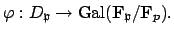Theorem 14.1.5   The homomorphism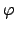is surjective.

Proof. Let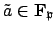be an element such that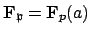. Lift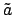to an algebraic integer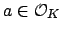, and let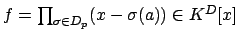be the characteristic polynomial of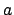over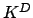. Using Proposition 14.1.4 we see that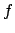reduces to the minimal polynomial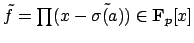of(by the Proposition the coefficients of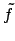are in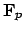, andsatisfies, and the degree ofequals the degree of the minimal polynomial of). The roots ofare of the form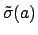, and the element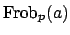is also a root of, so it is of the form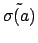. We conclude that the generator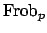of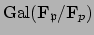is in the image of, which proves the theorem.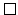Definition 14.1.6 (Inertia Group)   The is the kernel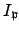of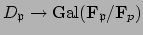.

Combining everything so far, we find an exact sequence of groups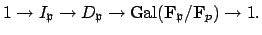(14.1)

The inertia group is a measure of how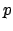ramifies in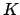.

Corollary 14.1.7   We have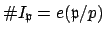, where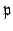is a prime ofover.

Proof. The sequence (14.1.1) implies that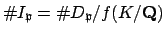. Applying Propositions 14.1.3-14.1.4, we have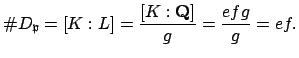Dividing both sides by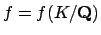proves the corollary.We have the following characterization of.

Proposition 14.1.8   Let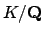be a Galois extension with group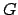, letbe a prime lying over a prime. Then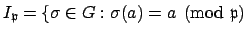for all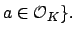Proof. By definition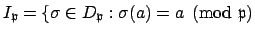for all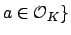, so it suffices to show that if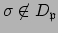, then there existssuch that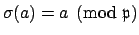. If, we have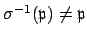, so since both are maximal ideals, there exists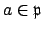with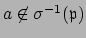, i.e.,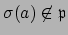. Thus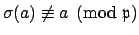.Figure 14.1.2 is a picture of the splitting behavior of a prime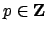.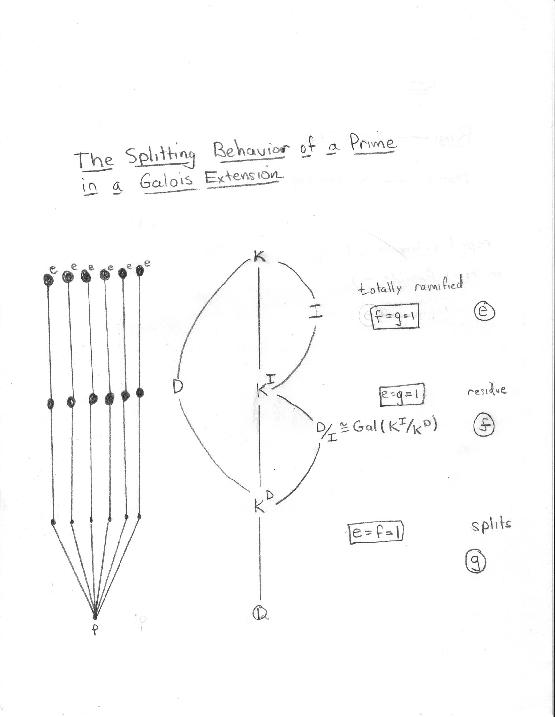William Stein 2004-05-06